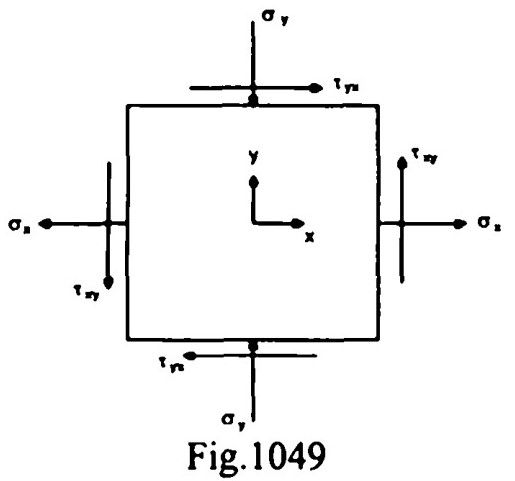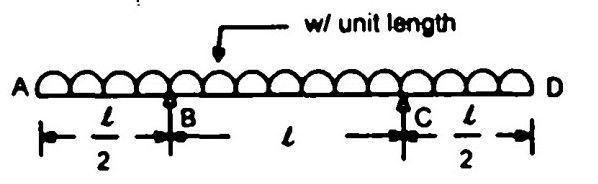top of pageSearch

# Strength of Materials(261-280)

Updated: Apr 29, 2020

261. If the eccentricity of total self weight ' W' of a masonry dam at its base is equal to one fourth of the base width ' B '. then the maximum pressure at the base is given by

262. The rectangular column shown in Fig.10.47 carries a load 'P' having eccentricity and e along the x-axis and y-axis respectively.The stress at any point (x, y) is given by263.Which one of the following pairs is correctly matched ?

Strain hardening material -Stiffening effect felt at some stage Orthotropic material-Different properties in three perpendicular directions Isotropic material-Same physical property in all directions at a point

264. In a plane stress problem there are normal tensile stresses 𝜏 and 𝜏accompanied by shear stress 𝜏ₓᵧ at a point along orthogonal cartesian coordinates x and y respectively. If it is observed that the minimum principal stress on a certain plane is zero then265. In an experiment it is found that the bulk modulus of a material is equal to its shear modulus. The Poisson's ratio is 0.125

266. A mild steel bar is in two parts having equal lengths. The area of cross section of Part-1 is double that of Part-2. If the bar carries an axial load 'P' then the ratio of elongation in Part-1 to that in part-2 will be 1/2

267. At a point in a steel member, the major principal stress is 200 MPa (tensile) and the minor principal stress is compressive. If the uniaxial tensile yield stress is 250 MPa , then according to the maximum shear stress theory, the magnitude of the minor principal stress (compressive) at which yielding will commence is 50 Mpa

268. The limit of proportionality of a certain sample is 300 MPa in simple tension. It is subjected to principal stresses of 150 MPa (tensile), 60 MPa (tensile) and 30

MPa (tensile). According to the maximum principal stress theory, the factor of safety in this case would be 2

269. Shear centre of a semi circular arc strip of radius 'r' will be at a distance of X from the centre of the arc, where 'X' is equal to 4r/π

270. A round bar made of same material consists of 3 parts each of 100 mm length

having diameters of 40 mm, 50 mm, and 60 mm respectively. If the bar is subjected to an axial load of 10 kN, the total elongation of the bar would be (E is

modulus of elasticity in kN/mm )271.If a member is subjected to tensile stress of px, compressive stress of py and tensile stress of pz along the x, y, and z directions respectively, then the resultant strain ex' along the V direction would be (E is Young's modulus of elasticity, μ is

Poisson's ratio)272. A cylindrical bar of 20 mm diameter and 1 m length is subjected to a tensile test. Its longitudinal strain is 4 times that of its lateral strain. If the modulus of elasticity is 2 x 10 N/mm², then its modulus of rigidity will be

0.8 x 10 N/mm²

273. The rectangular block shown in Fig. 10.48 is subjected to pure shear of intensity 'q'If BE represents the principal plane and the principal stresses are σ₁, σ₂ ; then the

values of θ, σ₁ and σ₂ will be respectively. 45°, 135°; + q and-q

274. The state of stresses on an element is shown in the Fig. 10.49. The values of

stresses are ox (=32 MPa); ay (=-10 MPa) and major principal stress ot (= 40 MPa)The minor principal stress 'σ2' is -18 MPa

275.A material of Young's modulus 'E' and Poisson's ratio 'μ' is subjected to two

principal stress σ and σ at a point in a two-dimensional stress system. The strain energy per unit volume of the material is276. Given that for a channel section, the width of flange = b, the depth of the web

between centres of flanges = h, the thickness of flange = t, the moment of

inertia =1, the distance V of the shear centre outside the channel section from

the mid thickness of the web is th²b²/4I

277. A simply supported beam is shown in Fig. 10.50.The corresponding SFD and BMD would be278. The beam ABC shown in Fig. 10.52 is horizontalThe distance to the point of contraflexure from the fixed end 'A' is

0.333

279. A beam's S.F.D. and B.M.D. are shown in Fig. 10.53(a) and (b) respectively.The corresponding load diagram will be280.The bending moment diagram of the beam shown in Fig is